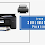### Problem

Given a string, find the longest substring that contains only two unique characters. For example, given "abcbbbbcccbdddadacb", the longest substring that contains 2 unique character is "bcbbbbcccb".

### JavaScript Code

```function maxSubStringKUniqueChars(s, k) {
//declare a counter
var map = {};
var start = 0;
var maxLen = 0;
var maxSubstring = null;

for (var i = 0; i < s.length; i++) {
//add each char to the counter
var c = s.charAt(i);
if(map[c]!=null){
map[c] = map[c]+1;
}else{
map[c] = 1;
}

if(Object.keys(map).length == k+1){
//get maximum
var range = i-start;
if(range > maxLen){
maxLen = range;
maxSubstring = s.substring(start, i);
}

//move left cursor toward right, so that substring contains only k chars
var first = s.charAt(start);
while(Object.keys(map).length > k){
var count = map[first];
if(count>1){
map[first] = count-1;
}else{
delete map[first];
}
start++;
}
}
}

if (Object.keys(map).length == k && maxLen == 0) {
return s;
}

return maxSubstring;
}
console.log(maxSubStringKUniqueChars('abcabcbb', 3));
```

1.Longest substrate is the basic and valued concern of this blog and we should read this blog. After reading this blog the readers will understand different concepts on this site https://www.writessays.biz/. This blog is an ideal blog in this way of blogging and creative ideas.

2.## BlogrollSrinivas Dasari find me on facebook follow me on twitter